# Algebra 1 : Factoring Polynomials

## Example Questions

### Example Question #41 : Factoring Polynomials

Find the GCF (greatest common factor) of the following polynomial expression.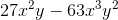Possible Answers: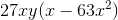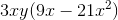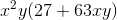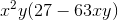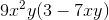Correct answer:Explanation:

Let's find the GCF of the coefficients first.  Remember, "coefficent" means the constant, the number to the left of the variables.

The first term has "27" and the second term has "-63" as their coefficients.  The GCF of 27 and -63 is 9.  9 is the largest number that can divide BOTH 27 and -63 without leaving a remainder.

Now, for the variables.  The first term has two "x" variables and one "y" variable.  The second term has three "x" variables and two "y" variables.  The greatest amount of x's and y's that the terms share is two x's and one y.

So, the GCF of the expression is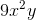.  When you divide the terms by this, you get:### Example Question #41 : How To Factor A Polynomial

Find the GCF (greatest common factor) of the following polynomial expression.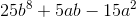Possible Answers: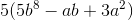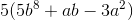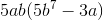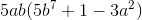Correct answer:Explanation:

Let's first look at the coeffiecients of these three terms.  They are: 25, 5, and -15.  The GCF of these three numbers is 5, because 5 is the largest number that can divide all of them without leaving a remainder.

So, "5" is the first part of our GCF.

Next, let's look at the variables.  We have two types, "a" and "b".  The first term has eight "b" variables, the second term has one "a" and one "b" variable, and the third term has two "a" variables.

THERE IS NO COMMON FACTOR FOR VARIABLES!

The first term has no "a" variables, and the third term has no "b" variables.  They have no variables in common.  So, we cannot factor out the variables.

The GCF of the polynomial is simply "5".  When we divide each term by 5, we get:### Example Question #42 : How To Factor A Polynomial

Find the GCF (greatest common factor) of the following polynomial expression.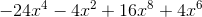Possible Answers: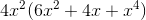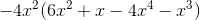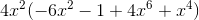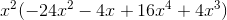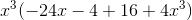Correct answer:Explanation:

Let's first find the GCF of the coefficients.  The coefficients of the four terms are -24, -4, 16, and 4.  The biggest number that can divide ALL of them without leaving a remainder, is 4.

The first part of our GCF is "4."

Next, let's look at the variables.  We have only one type, "x."  The first term has four "x" variables, the second has two, the third has eight, and the fourth has six.

The greatest amount of "x" variables that each term has in common is 2.  Each has at least two x variables.  The 1st, 3rd, and 4th terms have MORE than two x variables, but since the 2nd term only has two x's, that's the greatest amount that we can factor from ALL of the terms.

So, our GCF is 4x^2.  When we divide each of the terms by 4x^2, we get:### Example Question #42 : How To Factor A Polynomial

Find the GCF (greatest common factor) of the following polynomial expression.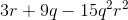Possible Answers: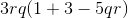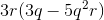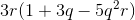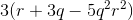Correct answer:Explanation:

Let's find the GCF of the coefficients, first.  Our coefficients are 3, 9, and -15.  The GCF is 3.  3 is the biggest number that can divide EACH of the numbers without leaving a remainder.

So, 3 is the first part of our GCF.

Next, look at the variables.  THERE IS NO COMMON VARIABLE!  The first term has an "r" variable, but the second term only has a "q" variable.  They do not have anything in common, so we cannon factor out any variables.

So, the only factor all of the terms have in common is "3," and when you divide each of the terms by 3, we get:### Example Question #42 : How To Factor A Polynomial

Factor the following polynomial expression completely.  Use the "factor-by-grouping" method.

(Method is demonstrated in the answer explanation)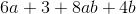Possible Answers: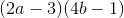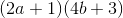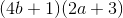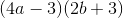Correct answer:Explanation:

At first, it looks like none of the the terms have any factors in common.  But here's how we factor by "grouping":  we separate the four terms into two groups, with two terms in each group.  Then, we find the GCF of each separate group.

First group: 6a + 3

Second group: 8ab + 4b

Start with the first group.  The GCF is "3," since that's the biggest number that can divide each term without leaving a remainder.  So, group 1 is: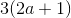Second group.  The GCF is 4b.  Check it, if you're not sure.  That means that when group 2 is factored, we get: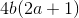So, since both group 1 and group 2 were being ADDED to each other in our original expression, we can simply add the two factored groups to each other: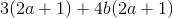The expression is still the same, we've just rewritten it in factored form.  If you multiply through the parentheses, you'll see that we still have the same original expression:But now, notice that the two factored terms have something in common!  They each have the factor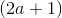So, we can divide (2a+1) from both terms, leaving us with:### Example Question #363 : Polynomials

Factor the following polynomial expression completely, using the "factor-by-grouping" method.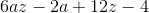Possible Answers: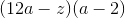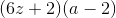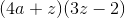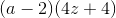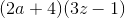Correct answer:Explanation:

Group the first two terms together: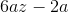Find the GCF: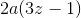Group the second two terms together: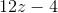Find the GCF: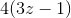The two factored terms both have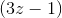as their GCF.

So, factoring outfrom each term gives us:### Example Question #21 : Factoring Polynomials

Which of the following expressions is a factor of this polynomial: 3x² + 7x – 6?

Possible Answers:

(3x + 2)

(x – 3x)

(x – 3)

(3x – 6)

(x + 3)

Correct answer:

(x + 3)

Explanation:

The polynomial factors into (x + 3) (3x - 2).

3x² + 7x – 6 = (a + b)(c + d)

There must be a 3x term to get a 3x² term.

3x² + 7x – 6 = (3x + b)(x + d)

The other two numbers must multiply to –6 and add to +7 when one is multiplied by 3.

b * d = –6 and 3d + b = 7

b = –2 and d = 3

3x² + 7x – 6 = (3x – 2)(x + 3)

(x + 3) is the correct answer.

### Example Question #41 : Factoring Polynomials

Factor the polynomial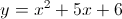.

Possible Answers:

y = (x + 3)(x + 2)

y = (x + 5)(x + 1)

y = (x – 2)(x + 3)

y = (x – 3)(x + 2)

y = (x + 6)(x + 1)

Correct answer:

y = (x + 3)(x + 2)

Explanation:

The product of the last two numbers should be 6, while the sum of the products of the inner and outer numbers should be 5x. Factors of six include 1 and 6, and 2 and 3. In this case, our sum is five so the correct choices are 2 and 3. Then, our factored expression is (x + 2)(x + 3). You can check your answer by using FOIL.

y = x2 + 5x + 6

2 * 3 = 6 and 2 + 3 = 5

(x + 2)(x + 3) = x2 + 5x + 6

### Example Question #31 : Factoring Polynomials

Factor: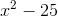Possible Answers:

The expression cannot be factored.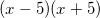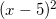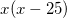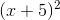Correct answer:Explanation:

Because both terms are perfect squares, this is a difference of squares: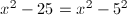The difference of squares formula is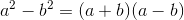.

Here, a = x and b = 5.  Therefore the answer is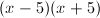.

You can double check the answer using the FOIL method: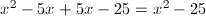### Example Question #361 : Polynomials

Factor: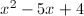Possible Answers: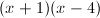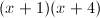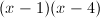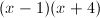Correct answer:Explanation:

The solutions indicate that the answer is: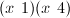and we need to insert the correct addition or subtraction signs. Because the last term in the problem is positive (+4), both signs have to be plus signs or both signs have to be minus signs. Because the second term (-5x) is negative, we can conclude that both have to be minus signs leaving us with:### All Algebra 1 Resources